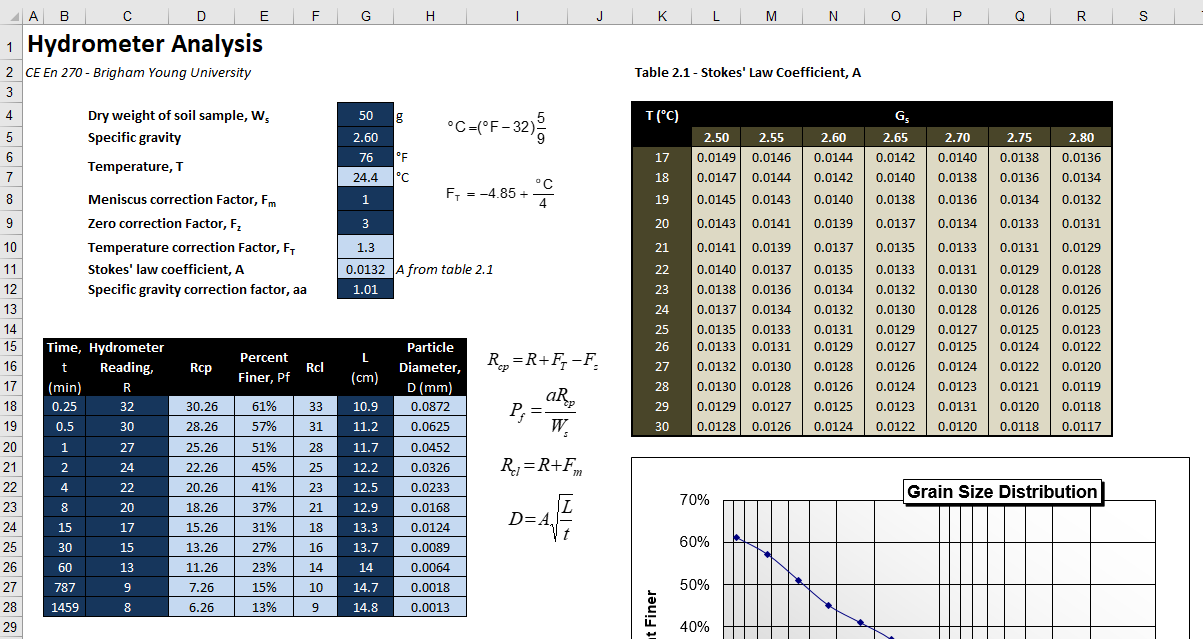# HW#6 - Hydrometer Analysis

A hydrometer analysis is a test done on a soil sample to determine the particle size distribution for the smaller fraction of the soil particles. It involves mixing the soil with water in a glass beaker and measuring how rapidly the particles settle out of the solution. The density of the solution changes with time as the particles settle to the bottom of the beaker. The following spreadsheet is designed to analyze the results of a hydrometer test:a. Before entering the formulas, first name the cells at the top of the spreadsheet as follows. Use these names in your formulas.

 Variable Cell Name Dry weight of sample G4 Ws Specific gravity G5 Gs Temperature (oF) G6 Tf Temperature (oC) G7 Tc Meniscus correction factor (Fm) G8 Fm Zero correction factor (Fz) G9 Fz Temperature correction factor G10 Ft Stoke's law coefficient G11 A Specific gravity correction factor G12 aa GS index list K5:R5 gslist Stoke's Law coeff. table K6:R19 stokestable

b. Enter a formula in cell G7 to convert the temperature from  oF to oC.

c. Enter a formula in cell G10 to compute the temperature correction factor (FT).

d. Enter a formula in cell G11 to find Stoke's law coefficient (A) by referenceing Table 2.1. To use this table, you will need to do a "double lookup". You will use a regular VLOOKUP to find the row with the right temperature in the first column using a range lookup. But then you will need to figure out which column should be used to return a value based on the Gs value (third argument to VLOOKUP). To do this you will pass the Gs value (in cell G5) to the MATCH function and use the gslist (K5:R5) to return the index (1,2,3,etc) corresponding to the column in the list where the match is found. In other words, you will embed a call to the MATCH function within the VLOOKUP function (you can assume that you will find an exact match for Gs with the MATCH function). This process is described in the "Two-Dimensional Lookup" section of the VLOOKUP chapter of the VBA Primer. (*Note: the example given in the primer is more complicated than this one. You will not need to use the TEXT() function.)

e. Enter the formula for the Rcp column.

f.  Enter the formula for the Percent finer (Pf) column.

g.  Enter the formula for the Rcl column.

h.  Enter the formula for the particle diameter (D) column. You do not need to do any units conversion. The A coefficient is calibrated to return a diameter in mm.